Next: Example Up: Number of roots of Previous: Mathematical background   Contents

Implementation

The purpose of the implementation is first to convert the trigonometric equation in sine and cosine of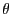: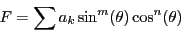(4.2)

into a polynomial in either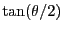or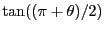. A first procedure return an upper bound of the degree of the resulting polynomial:

int Degree_Max_Convert_Trigo_Interval(int n,VECTOR &A,INTEGER_VECTOR &SSin,INTEGER_VECTOR &CCos);
int Degree_Max_Convert_Trigo_Interval(int n,INTEGER_VECTOR &A,
INTEGER_VECTOR &SSin,INTEGER_VECTOR &CCos);
with:
• n: number of terms of the equation
• A: the coefficients of each term which may be either real or integer
• Ssin: the sine power of each term
• Ccos: the cosine power of each term
Then we may use a procedure which compute the coefficients of the polynomial equation using the following procedures:

VOID Convert_Trigo_Interval(int n,VECTOR &A,INTEGER_VECTOR &SSin,
INTEGER_VECTOR &CCos,VECTOR &Coeff,int *degree);
VOID Convert_Trigo_Interval(int n,INTEGER_VECTOR &A,INTEGER_VECTOR &SSin,
INTEGER_VECTOR &CCos,INTEGER_VECTOR &Coeff,int *degree);
VOID Convert_Trigo_Interval(int n,INTERVAL_VECTOR &A,INTEGER_VECTOR &SSin,
INTEGER_VECTOR &CCos,INTERVAL_VECTOR &Coeff,int *degree);
with:
• n: number of terms of the equation
• A: the coefficients of each term which may be either real or integer
• Ssin: the sine power of each term
• Ccos: the cosine power of each term
• Coeff: the coefficients of the polynomial
• degree: the final degree of the polynomial
The previous procedures use the substitution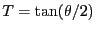which is not valid for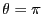. In that case we may use instead the substitution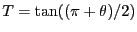and the coefficient of the resulting polynomial may be determined using the following procedures:

VOID Convert_Trigo_Pi_Interval(int n,VECTOR &A,INTEGER_VECTOR &SSin,
INTEGER_VECTOR &CCos,INTEGER_VECTOR &Coeff,int *degree);
VOID Convert_Trigo_Pi_Interval(int n,INTEGER_VECTOR &A,INTEGER_VECTOR &SSin,
INTEGER_VECTOR &CCos,INTEGER_VECTOR &Coeff,int *degree);
VOID Convert_Trigo_Pi_Interval(int n,INTERVAL_VECTOR &A,INTEGER_VECTOR &SSin,
INTEGER_VECTOR &CCos,INTEGER_VECTOR &Coeff,int *degree);
Similar procedures exists for interval trigonometric equations i.e. equations where the coefficients A are intervals. In that case degree will no more an integer but an INTERVAL which indicate the lowest and highest degree of the resulting polynomial. In some case the number of roots of the trigonometric equation may exceed the degree of the equivalent polynomial. For example the equation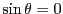has the roots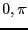while the degree of the equivalent polynomial is only 1. In all cases the total number of roots of the trigonometric equation will never exceed the degree+2.

Having determined the equivalent polynomial you my use the tools described in section 5 for determining the number of roots of the trigonometric equation. But you still have to manage the search interval. The following procedure is able to determine this search interval and to determine the number of roots of the trigonometric equation:

int Nb_Root_Trigo_Interval(int n,VECTOR &A,INTEGER_VECTOR &SSin,
INTEGER_VECTOR &CCos,REAL Inf,REAL Sup)
with:
• n: number of terms of the equation
• A: the coefficients of each term which may be either real or integer
• Ssin: the sine power of each term
• Ccos: the cosine power of each term
• Inf: the lower bound of the search interval
• Sup: the upper bound of the search interval
On success this procedure returns a number greater or equal to 0 and returns -1 if it has failed. Failure occurs either if the equation is equal to 0 or if Sturm method failed to determine the number of roots of the equivalent polynomial equation (this will happen if Inf or Sup are exact root of the equation).Next: Example Up: Number of roots of Previous: Mathematical background   Contents
Jean-Pierre Merlet 2012-12-20